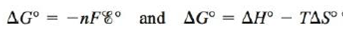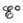Chapter 18, Problem 141CP

Chapter
Section
Textbook Problem

# Combine the equationsto derive an expression foras a function of temperature. Describe how one can graphically determine ∆H° and ∆S° from measurements ofat different temperatures, assuming that ∆H° and ∆S° do not depend on temperature. What property would you look for in designing a reference half-cell that would produce a potential relatively stable with respect to temperature?

Interpretation Introduction

Interpretation:

Two different equations showing the change in Gibbs free energy are given. The derivation of E° as a function of temperature for the given equations, the graphical determination of ΔH° and ΔS° from measurements of E° at different temperature and the property used for designing a reference half-cell that would produce a potential relatively stable with respect to temperature is to be stated.

Concept introduction:

Gibbs free energy is basically the maximum amount of non-expansion work done. Therefore, it is represented as,

Wmax=ΔG°

The relationship between Gibbs free energy change and cell potential is given by the formula,

ΔG°=nFE°cell

The relation between ΔG° , ΔH° and ΔS° is given as,

ΔG°=ΔH°TΔS°

To determine: The derivation of E° as a function of temperature for the given equations, the graphical determination of ΔH° and ΔS° from measurements of E° at different temperatures and the property used for designing a reference half-cell that would produce a potential relatively stable with respect to temperature.

The relation obtained from the given equations is,

E°cell=T(ΔS°nF)+(ΔH°nF)

Explanation

The relationship between ΔG° , ΔH° and ΔS° is given as,

ΔG°=ΔH°TΔS° (1)

Where,

• ΔG° is the standard Gibbs free energy change.
• ΔH° is the standard enthalpy change.
• ΔS° is the standard entropy change.
• T is the temperature at which reaction occurs.

The relationship between Gibbs free energy change and cell potential is given by the formula,

ΔG°=nFE°cell (2)

Where,

• ΔG is the Gibbs free energy change at the given temperature conditions.
• n is the number of moles of electrons that are involved in the reaction.
• F is the Faraday’s constant (96,485C/molee) .
• Ecell is the cell potential at the given temperature conditions.

Combine equation (1) and (2) as,

nFE°cell=ΔH°TΔS°

Rearrange the equation to obtain the value of E°cell as,

E°cell=T(ΔS°nF)+(ΔH°nF) (3)

When the graph between E°cell and T is plotted, it leads to a straight line and the value of ΔH° and ΔS° is obtained from the obtained straight line equation.

The relationship between ΔG° , ΔH° and ΔS° is given as,

ΔG°=ΔH°TΔS° (1)

Where,

• ΔG° is the standard Gibbs free energy change.
• ΔH° is the standard enthalpy change.
• ΔS° is the standard entropy change.
• T is the temperature at which reaction occurs.

The relationship between Gibbs free energy change and cell potential is given by the formula,

ΔG°=nFE°cell (2)

Where,

• ΔG is the Gibbs free energy change at the given temperature conditions

### Still sussing out bartleby?

Check out a sample textbook solution.

See a sample solution

#### The Solution to Your Study Problems

Bartleby provides explanations to thousands of textbook problems written by our experts, many with advanced degrees!

Get Started